### Home > A2C > Chapter Ch11 > Lesson 11.3.4 > Problem11-173

11-173.
1. Simplify each expression. Assume the denominator does not equal zero. Homework Help ✎

1.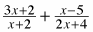2.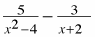3.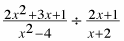4.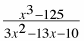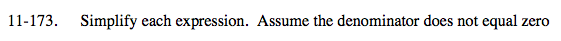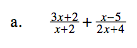Find the least common denominator.

$\frac{3x+2}{x+2}+\frac{x-5}{2(x+2)}$

$\frac{2(3x+2)}{2(x+2)}+\frac{x-5}{2(x+2)}$

$\frac{7x-1}{2x+4}$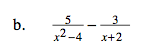Factor and then find the least common denominator.

$\frac{-3x+11}{x^2-4}$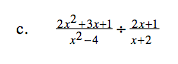Use the reciprocal to write the expression as a product, then factor and eliminate the common factors.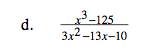Factor, then eliminate the common factors.